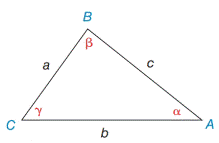Chapter 11.4, Problem 5EElementary Geometry For College St...

7th Edition
Alexander + 2 others
ISBN: 9781337614085

Solutions

Chapter
SectionElementary Geometry For College St...

7th Edition
Alexander + 2 others
ISBN: 9781337614085
Textbook Problem

In Exercises 5 and 6, state the form of the Law of Cosines used to solve the problem. Using the values provided, give the answer in a form such as a 2 = b 2 + c 2 − 2 a b   cos α . See the figure for Exercises 1 to 8.a) Find c if it is known that a = 5.2 ,   b = 7.9  and  γ = 83 ∘ . b) Find α if it is known that a = 6 ,   b = 9 ,  and  c = 10 .Exercises 1-8

To determine

a.

To Find:

The side of c by using Law of Cosines.

Explanation

Formula:

The Law of Cosines

a2=b2+c22bccosα

b2=a2+c22accosβ

c2=a2+b22abcosγ

Where α, β, and γ is the acute angle of the triangle, and a, b, and c is the sides of the triangle.

Calculation:

Given,

In triangle ABC,

a=5.2

b=7

To determine

b.

To find:

The angle of α by using the Law of Cosines.

Still sussing out bartleby?

Check out a sample textbook solution.

See a sample solution

The Solution to Your Study Problems

Bartleby provides explanations to thousands of textbook problems written by our experts, many with advanced degrees!

Get Started

Differentiate. H(u)=(uu)(u+u)

Single Variable Calculus: Early Transcendentals, Volume I

Explain the difference between a matched-subjects design and a repeated-measures design.

Essentials of Statistics for The Behavioral Sciences (MindTap Course List)

Multiply: 70601300

Elementary Technical Mathematics

Solve the equations in Exercises 112 for x (mentally, if possible). x+1=3x+1

Finite Mathematics and Applied Calculus (MindTap Course List)

For

Study Guide for Stewart's Multivariable Calculus, 8th